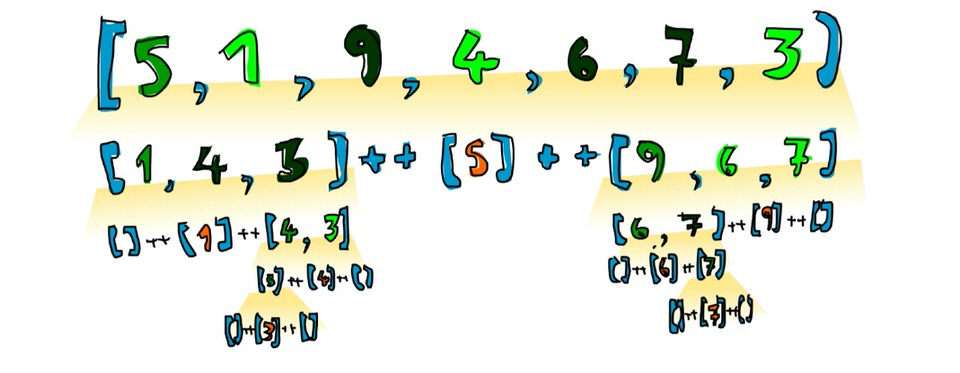Swift Quick Sort Algorithm With Recursion and Generics
Aug 22, 2016

# Summary

There are many sorting algorithm and many way to implement it. Quicksort is one of the quickest.

Today we will learn how to implement quicksort in Swift with generic and protocol conformance.

# Motivation

I was reading Learn You a Haskell book today and one of the example of recursion chapter was quicksort. It just tickled me to implement the same thing in Swift.

Great book by the way, you should consider this Joy of coding bundle for more books.

# Sorting numbers

Lets say we have this array of jumbled Int that we wanted to sort

``````let arrNum = [6, 4, 7, 9, 22, 78, 11, 1, 0, 234]
``````

Now lets define our function interface

``````func quickSort(array: [Int]) -> [Int] {
return array
}
``````

That codeblock will just return the unsorted array, which isn’t what we wanted. So lets get it working.

``````func quickSort(array: [Int]) -> [Int] {
if array.isEmpty { return [] } // 1

let first = array.first! // 2

let smallerOrEqual = array.dropFirst().filter { \$0 <= first } // 3
let larger         = array.dropFirst().filter { \$0 > first } // 4

return quickSort(smallerOrEqual) + [first] + quickSort(larger) // 5
}
``````
1. Every recursive function needs an escape scenario, else it would go to an infinite loop. In this case, we want the recursion to break when the array that is passed is empty.
2. We need to store the first element of the array to compare it with the smaller or larger number.
3. The first half of quicksort is all the values that are smaller or equal to our first number. We can filter the array after we drop the first value.
4. The second half is all the values that are larger than our first number.
5. The first and second half would then be recursed and added to the first value.

This graphic from Learn You a Haskell explains the concept perfectly:Let’s test it!

``````quickSort(arrNum) // [0, 1, 4, 6, 7, 9, 11, 22, 78, 234]
``````

Yay! It’s working!

# Generics

As it is now, the function only works for `Int` type. What do we do if we want to sort a `Double` array?

We can either copy paste the whole function and just change the type to `Double` like so:

``````func quickSort(array: [Double]) -> [Double] {
// implementation
}
``````

But then we will have to copy paste it again if we need to compare `String`, `Float` or other types.

There must be a better way. And yes there is! We can use Generics

``````func quickSort<T: Comparable>(array: [T]) -> [T] {
if array.isEmpty { return [] }

let first = array.first!

let smallerOrEqual = array.dropFirst().filter { \$0 <= first }
let larger         = array.dropFirst().filter { \$0 > first }

return quickSort(smallerOrEqual) + [first] + quickSort(larger)
}
``````

As you can see, the implementation of the method doesn’t change. Only the interface and type is changed.

Our function will now be able to sort any types that conforms to the `Comparable` protocol. Swift already implemented this for most of the primitive types that we encounter in daily basis.

Let’s test it:

``````let arrStr = ["a", "x", "R", "hey", "c", "Jon Snow"]
let arrDouble = [1.4, 6.7, 9.2, 6.4, 1.4]

quickSort(arrNum)    // [0, 1, 4, 6, 7, 9, 11, 22, 78, 234]
quickSort(arrStr)    // ["Jon Snow", "R", "a", "c", "hey", "x"]
quickSort(arrDouble) // [1.4, 1.4, 6.4, 6.7, 9.2]
``````

It’s working again! However it wouldn’t work if Swift isn’t able to infer the type of the array.

``````let emptyInt = []
genericQuickSort(emptyInt)
// error: 'NSArray' is not convertible to '[_]'; did you mean to use 'as!' to force downcast?
``````

We can fix it by explicitly defining the type:

``````let emptyInt: [Int] = []
quickSort(emptyInt) // []
``````

Now Swift compiler wouldn’t complain and will return an empty array as expected!

# Comparable conformance

Our generic quickSort() will be able to sort anything that conforms to `Comparable` protocol. But it wouldn’t be able to sort a custom struct. For example:

``````struct Dog {
let id: Int
let name: String
}

let dog1 = Dog(id: 19123, name: "Bella")
let dog2 = Dog(id: 26234, name: "Ollie")
let dog3 = Dog(id: 31241, name: "Klue")

quickSort([dog2, dog1, dog3])
// error: cannot convert value of type '[Dog]' to expected argument type '[_]'
``````

This happens because Swift doesn’t know how to sort a custom struct that doesn’t conform to `Comparable`. How do we fix it? By conforming it to `Comparable` of course!

``````struct Dog: Comparable { // 1
// definition
}

func < (l: Dog, r: Dog) -> Bool { // 2
return l.id < r.id
}

func == (l: Dog, r: Dog) -> Bool { // 3
return l.id == r.id
}
``````

Conforming to `Comparable` is as easy as 1-2-3

1. Conform the custom class or struct to `Comparable`
2. Implement the `<` operation by comparing a property of the struct that already is comparable
3. Do the same thing with `==` operation

Step 2 and 3 can be as simple as comparing ID to as complex as comparing all of the properties in the struct. It depends on your needs.

Now let’s test it again

``````quickSort([dog2, dog1, dog3])
// [Dog(id: 19123, name: "Bella"), Dog(id: 26234, name: "Ollie"), Dog(id: 31241, name: "Klue")]
``````

It’s working!

# Conclusion

It is very easy to implement the Swift version of recursive quicksort with generics. The type inference system helps us a lot.

# Where to go from here?

Now that you can sort a randomized array, how about implementing a recursive binary sort with Swift?

I will post the answer on future blog post.